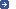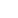– the intergovernmental organization through which Member States act together
on matters related to measurement science and measurement standards.
 Search facility:| Site map | News | Contact us> You are here: worldwide metrology: committee structure > CIPM > 1946CIPM, 1946: Resolution 2 Version françaiseDefinitions of electric units*... (A) Definitions of the mechanical units which enter the definitions of electric units: Unit of force - The unit of force [in the MKS (metre, kilogram, second) system] is the force which gives to a mass of 1 kilogram an acceleration of 1 metre per second, per second. Joule (unit of energy or work) - The joule is the work done when the point of application of 1 MKS unit of force [newton] moves a distance of 1 metre in the direction of the force. Watt (unit of power) - The watt is the power which in one second gives rise to energy of 1 joule. (B) Definitions of electric units. The Comité International des Poids et Mesures (CIPM) accepts the following propositions which define the theoretical value of the electric units: Ampere (unit of electric current) - The ampere is that constant current which, if maintained in two straight parallel conductors of infinite length, of negligible circular cross-section, and placed 1 metre apart in vacuum, would produce between these conductors a force equal to 2 ´ 10-7 MKS unit of force [newton] per metre of length. Volt (unit of potential difference and of electromotive force) - The volt is the potential difference between two points of a conducting wire carrying a constant current of 1 ampere, when the power dissipated between these points is equal to 1 watt. Ohm (unit of electric resistance) - The ohm is the electric resistance between two points of a conductor when a constant potential difference of 1 volt, applied to these points, produces in the conductor a current of 1 ampere, the conductor not being the seat of any electromotive force. Coulomb (unit of quantity of electricity) - The coulomb is the quantity of electricity carried in 1 second by a current of 1 ampere. Farad (unit of capacitance) - The farad is the capacitance of a capacitor between the plates of which there appears a potential difference of 1 volt when it is charged by a quantity of electricity of 1 coulomb. Henry (unit of electric inductance) - The henry is the inductance of a closed circuit in which an electromotive force of 1 volt is produced when the electric current in the circuit varies uniformly at the rate of 1 ampere per second. Weber (unit of magnetic flux) - The weber is the magnetic flux which, linking a circuit of one turn, would produce in it an electromotive force of 1 volt if it were reduced to zero at a uniform rate in 1 second.Reference:Procès-Verbaux des Séances du CIPM (1946), p. 129Note: The definitions contained in this Resolution were ratified in 1948 by the 9th CGPM, which also adopted the name newton (Resolution 7) for the MKS unit of force.SummaryConsultative CommitteesCIPM publicationsJoint Working Groupsad hoc Working Group on GovernanceStrategic planning: BIPM and Consultative CommitteesCIPM Sub-Committees and ad hoc GroupsCIPM election processMembers of the CIPMPhotographs of the CIPMCIPM Decisions and RecommendationsBIPM - Pavillon de Breteuil F-92312 Sèvres Cedex FRANCE Disclaimer Privacy Policy Copyright © BIPM all rights reserved